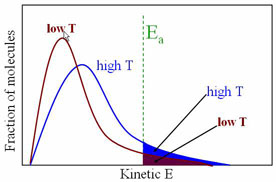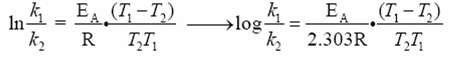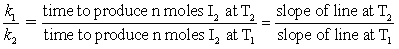Effect of Temperature on rate of Reaction

The rate of reaction is a function of many things, including surface area, concentration and temperature. For a reaction to take place, the molecuels must collide with enough energy (called the Activation Energy, EA) Consider the reactant molecules to be at a particular temperature. By definition, the molecules have an average kinetic energy, some with more than the average, some with less. If we increase the temperature of a system, the average kinetic energy will increase and the number of molecules with the 'minimum' energy to react, thus increasing the frequency (rate) of reaction.Svante Arrhenius has shown that for simple reactions, the equation

k = Ae-Ea/RT      ln k = -EA/RT + ln A

where k = rate constant, A is the collision frequency factor (function of the nature of reaction) R is the gas constant, T is the absolute temperature, EA is the activation energy, and e is the base of the natural logarithms. We want to know the relationship between rate of reactions and two different temperatures. After some creative algebra, we can transform the Arrehenius equation into something managable:k is the rate constant at temperature T, and k' is the rate at T'.
In this experiment, the reaction to be studied is the oxidation of HI to I2 by the action of H2O2 according to the equation

1 H2O2 + 2 HI  2 H2O + 1 I2

This reaction has been shown to be a second order reaction, first with respect to [HI], first with respect to [H2O2]. The experimentally determined rate law is of the form:

rate = k[HI][H2O2]

The total volume of the solution containing the components of the reaction is kept constant during the course of this experiment; as another simplification, conditions are adjusted so that the reaction follows pseudo first order kinetics. This is done by maintaining the concentration of [HI], so the rate law becomes

rate = k'[H2O2]  where k' = k[HI]

This reaction will thus behave is a pseudo first order kinetics under these conditions.

To calculate the activation energy Ea, we simply need to determine the ratio of the rate constants k1/k2 at two different temperatures T2 and T1. There exists many different ways of determining rate constants. Here, we will measure the time taken to produce identical amounts of I2 at two temperatures, T and T'. We will have a series of data that is amount of Na2S2O3 and time. If we graph this (amount of Na2S2O3 (x) vs. time(y) using a computer and getting the equation and R2) the slope will be how much time is required per mole of Na2S2O3. Since time and rate are inversely proportional we can obtain the rate constant ratios by:One experiment will be performed at temperature T2, and another at different temperature T1, using the same volumes and concentrations of reactants in both experiments. The time required to produce the same amount of I2 at each of the two temperatures will be measured and used to calculate k1/k2.

In this experiment, HI is produced by dissolving KI in a solution containing an excess of H2SO4:

1 H2SO4 + 1 KI  1 HI + KHSO4

Starch is added to this solution as the indicator of free iodine. We start the stopwatch at the moment we add our second reactant, H2O2. Of course the production of I2 begins immediately, and the blue color will instantly appear. A measured volume of 0.1 M Na2S2O3 is then added, causing the blue color to disappear due to the reduction of the I2 by thiosulfate. The solution will stay colorless until enough additional I2 is produced by H2O2-HI oxidation to react with the remaining Na2S2O3 in the solution. At this point the blue color will reappear. The time is noted. This represents the time required to produce I2 equivalent to the Na2S2O3 in the volume of 0.1 M Na2S2O3 which was added.

The entire reaction will be repeated with the same concentrations, but at a different temperature.

Procedure:

Chemicals needed- KI, 0.100 M Na2S2O3, 3% H2O2, starch solution, 6M H2SO4, ice

You will be running the entire reaction procedure twice, once in a water-ice bath at around 0.0 °C, and once at around 25 °C. The procedure below is the same for both runs.

1. Add  your particular temperature of water (room T water or ice water) to a 1L beaker and a 250 mL beaker with your particular temperature of water (room T water or ice water) The 1 L beaker with be a constant temperature bath for the smaller beaker, so it does not need that much.
2. Place a 400 mL beaker on the lab balance, and add 1.00 g of KI to it. It is important to get as close to 1.00 g as possible as you want the masses to be the same for both runs.
3. Dissolve the KI in about 250 mL of water. Add 15 mL of 6M H2SO4 (caution!) and carefully place the beaker in the 1000 mL water (ice?) bath. Place a stir bar in the solution and place the entire system on a stir plate and turn it on (no heat!!)
4. Make 50 mL of a 0.6% H2O2 solution (10 mL 3% H2O2 and 40 mL DI H2O) exact volumes are best.
5. Carefully measure out 5.0 mL of this solution and place it in a test tube, which is sitting in the 250 mL water bath beaker. Put a stopper on the test tube.
6. Rinse your buret twice and then fill it with 0.100 M Na2S2O3.
7. You want to wait until everything is at the desired temperature before you go any further. Once you start the reaction, you will want to keep the system stirring to keep everything at the same (water bath) temperature.
8. Position the 400 mL beaker (plus its water bath) under the buret. Record the temperature of the solution.
9. Add 4-6 drops of starch indicator to the 400 mL beaker.
10. Add the H2O2 to the solution and begin timing. The solution should immediately turn blue. That’s a good thing. The stopwatch does not get stopped until you have your (~12-20) measurements.
11. One student should be reading the buret and recording data (known as buret person), whilst the other watch the clock and the solution for the color change (clock person)
12. Buret person measures the volume in the buret. Then they add about 1 mL of solution and write down the new volume (how much was just added?) The solution should turn clear. At the instant the solution turns blue again, (clock person calls out the time, since they are watching the reaction) record the time (buret person).
13. Buret person adds 1 more mL, measures and records the new volume. The solution turns clear. When it turns blue again, measure and record the time.
14. Continue this procedure until you have added more than 20 mL of Na2S2O3 or until the blue color just stops coming back.

Once you have run the experiment twice, make the appropriate graphs, determine the slope, calculate the appropriate ratio of the rate constants, and calculate the activation energy of this reaction. When you are determining the slope, you should use only data that gives a linear shape. If your data has a logarithmic curve to it, use only the first 6-8 data points to determine the slope.

For this experiment, you are writing a short form memo lab report. You are ultimately trying to determine the activation energy of the oxidation reaction of HI to I2 by the action of H2O2. You must submit computer generated graphs of VNa2S2O3 vs. time. (where should they be?) There are no prelab questions, however if you want to start the lab, you will need to show the instructor your premade data sheet (recycled paper, of course) before you step foot in the lab. You also need to write up a flow-chart of the procedure. No data sheet or flow chart, no lab for you!